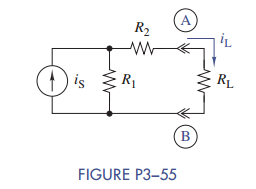Chat Now

# (a) Find the Thévenin or Norton equivalent circuit seen by RL in Figure P3−55. (b) Use the...

### (a) Find the Thévenin or Norton equivalent circuit seen by RL in Figure P3−55. (b) Use the...

(a) Find the Thévenin or Norton equivalent circuit seen by RL in Figure P3−55.

(b) Use the equivalent circuit found in part (a) to find iL in terms of iS, R1, R2, and RL.manish jayant 28-Jul-2020Get solution